Class 12 Chemistry NCERT Solutions for Chapter 7 The p-Block Elements is the best guide for the students appearing for boards and competitive exams. The solutions contain answers to the questions provided in the textbook. These help in strengthening all the concepts related to chapter 7 that help in better preparations.

NCERT Solutions not only help the students appearing in different boards but also the one’s appearing in the competitive exams. The students can practice the solutions provided by the subject experts.

 Board CBSE Textbook NCERT Class Class 12 Subject Chemistry Chapter Chapter 7 Chapter Name The p-Block Elements Number of Questions Solved 74 Category NCERT Solutions

## NCERT Solutions for Class 12 Chemistry Chapter 7 The p-Block Elements

Class 12 Chemistry Chapter 7 explains the p block elements and their properties, The elements in group 15, 16 and 17 are discussed along with their properties. Various concepts such as electronegativity, chemical and physical properties, ionization therapy, etc. are discussed in detail.

This chapter is important from examination perspective and the students are advised to go through the NCERT Solutions for better practice. The solutions are provided along with the diagrams for better understanding.

NCERT IN-TEXT QUESTIONS

Question 1.
Why are pentahalides more covalent than trihalides in the members of the nitrogen family?
The electronic configuration of the elements of nitrogen family (group 15) is ns2p3. Because of the inert pair effect, the valence s-electrons cannot be released easily for the bond formation. This means that the elements can form trivalent cation (E3+) by releasing valence p-electrons while it is difficult to form pentavalent cation (E5+). Under the circumstances, if all the five valence electrons are to be involved in the bond formation, the compounds showing pentavalency or +5 oxidation state must be of covalent nature. This is particularly the case in the higher members (Sb, Bi) of the family where the inert pair effect is quite prominent.

Question 2.
Why is BiH3 the strongest reducing agent amongst all the hydrides of Group 15 elements?
This is because as we move down the group, the size increases, as a result, length of E-H bond increases and its strength decreases, so that the bond can be broken easily to release H2 gas. Hence, BiH3 is the strongest reducing agent.

Question 3.
Why is N2 less reactive at room temperature?
In the nitrogen molecule (N2), two atoms of nitrogen are linked by triple bond (N = N). Due to small atomic size of the element (atomic radius = 70
pm), the bond dissociation enthalpy is very high (946 kJ mol1). This means that it is quite difficult to cleave or break the triple bond at room temperature. As a result, N2 is less reactive at room temperature.

Question 4.
Mention the conditions required for the maximum yield of ammonia.
In Haber’s process, ammonia is formed by the following reaction.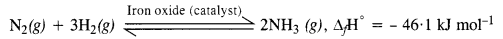According to Le-chatelier’s principle, the favourable conditions for the maximum yield of ammonia are :
(i) Low temperature : But optimum temperature of 700 K is necessary to keep the forward reaction in progress.
(ii) High pressure : Pressure to the extent of about 200 atm is required.
(iii) Catalyst & promoter : In order to achieve the early attainment of equilibrium, iron oxide acts as catalyst. Along with that; K2O, Al2O3 or Mo metal may act as the promoter to increase the efficiency of the catalyst.

Question 5.
How does ammonia react with blue solution having Cu2+ ions ?
When ammonia gas is passed through blue solution containing Cu2+ ions to give a soluble complex with deep blue colour.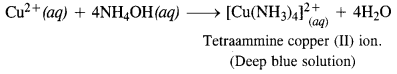Question 6.
What is the covalency of nitrogen in N2O5?
The covalent structure of nitrogen pentoxide (N2O5) is given. Since the nitrogen atom has shared four electron pairs, its covalency is four in the molecule of N2O5.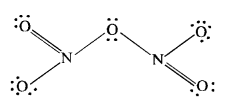Question 7.
Bond angle in PH4+ is higher than that in PH3. Why?
P in PH3 is sp3-hybridized with 3 bond pairs and one lone pair around P. Due to stronger lp-bp repulsions than bp-bp repulsions, tetrahedral angle decreases from 109°28′ to 93.6°. As a result, PH3 is pyramidal. In PH4+, there are 4 bp’s and no lone pair. As a result, there are only identical bp-bp repulsions so that PH4+ assumes tetrahedral geometry and the bond angle is 109°28′.Hence, bond angle of PH4+ > bond angle of PH3

Question 8.
What happens when white phosphorus is heated with concentrated NaOH solution in an inert atmosphere of CO2?
A mixture of sodium hypophosphite and phosphine gas is formed upon heating the reaction mixture in an inert atmosphere.Question 9.
What happens when PCl5 is heated?
Upon heating, PCl5 dissociates to give molecules of PCl3 and Cl2. Actually, the two P—Cl (a) bonds with more bond length break away from the molecule leaving three P— Cl(e) bonds attached to the central P atom since these are more firmly linked
PCl5 $$\underrightarrow { heat }$$ PCl3 + Cl2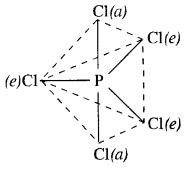Question 10.
Write the balanced equation for the hydrolytic reaction of PCl5 in heavy water.
With heavy water (D2O) ; phosphorus pentachloride (PCl5 reacts as follows :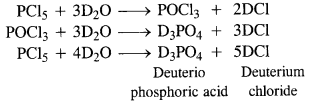Question 11.
What is the basicity of H3PO4 ?
The acid is tribasic since it has three P—OH bonds which can release H+ ions.

Question 12.
What happens when phosphorus acid (H3PO3) is heated ? (C.B.S.E. 2008)
In phosphorus acid (H3PO3), central atom P is in +3 oxidation state. Upon heating, it gives a mixture PH 3 (P in -3 oxidation state) and H3PO4 (P in +5 oxidation state). This means that phosphorus acid undergoes disproportionation reaction.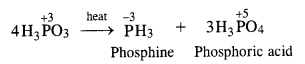Question 13.
List the important sources of sulphur.
Combined sulphur exists as sulphates, such as gypsum, epsom, baryte and sulphides such as galena, zinc blende, copper pyrites, etc. Traces of sulphur occur as hydrogen sulphide in volcanoes. Few organic materials like eggs, proteins, garlic, onion, mustard, hair and wool contain sulphur. 0.03 – 0.1% sulphur is present in the earth’s crust.

Question 14.
Write the order of the thermal stability of the hydrides of group 16 elements.
The order of thermal stability of hydride is :
H2O > H2S > H2Se > H2Te
This is related to the bond dissociation enthalpies of the E—H bonds where E stands for the element.
E—H bond :                                           O—H S—H Se—H Te—H
Bond dissociation enthalpy : (kJ mol-1) 463    347      276  238
Based on bond dissociation enthalpy, H2O is maximum stable thermally while H2Te is the least stable.

Question 15.
Why is H2O a liquid and H2S a gas?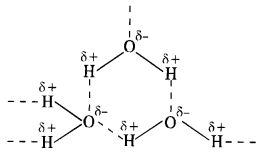In H2O, the electronegativity difference between 0(3·5) and H(2·1) is more than difference between S(2·5) and H(21) in H2S. As a result, O—H bond is more polar than S—H bond. This leads to inter molecular hydrogen bonding in H20 molecules while it is almost absent in the molecules of H2S. The H2O molecules get associated and consequently exist as liquid (water). The association in H2S molecules is negligible and it exists as a gas at room temperature.

Question 16.
Which of the following does not react with oxygen directly? Zn, Ti, Pt, Fe
Pt being a noble metal does not react with oxygen directly. In contrast, Zn, Ti and Fe are active metals and hence they react with oxygen directly to form their oxides.

Question 17.
Complete the following reactions :
(i) C2H4 + O2
(ii) Al + O2
(i) C2H4 + 3O2 $$\underrightarrow { heat }$$ 2CO2 + 2H2O
(ii) 4Al + 3O2 $$\underrightarrow { heat }$$ 2Al2O3

Question 18.
Why does O3 act as a powerful oxidising agent ? (C.B.S.E. 2013)
Upon heating, ozone (O3) readily decomposes to give molecular oxygen (O2) which is more stable along with nascent oxygen (O). The released nascent oxygen readily takes part in oxidation reactions. Therefore, ozone acts as a powerful oxidising agent.
O3 $$\underrightarrow { heat }$$ O2 + O (Nascent)

Question 19.
How is ozone estimated quantitatively ?
When ozone reacts with an excess of KI solution buffered with a borate buffer (pH = 9.2), iodine is liberated which can be titrated against standard solution of sodium thiosulphate. This is used as a method of estimation of ozone quantitatively.

Question 20.
What happens when sulphur dioxide gas is passed through an aqueous solution of Fe(III) salt ?
The gas is a reducing agent and reduces a Fe(III) salt to Fe(II) salt.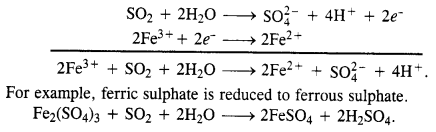Question 21.
Comment on the nature of two S—O bonds formed in SO2 molecule. Are the two bonds in the molecule equal ?
The two S—O bonds in the molecule are equal with bond length equal to 143 pm. This means that SO2 molecule exhibits two resonating structures as shown below.Question 22.
How is presence of SO2 detected ?
Ans.
Presence of SO2 is detected by bringing a paper dipped in acidified potassium dichromate near the gas. If the paper turns green, it shows the presence of SO2 gas.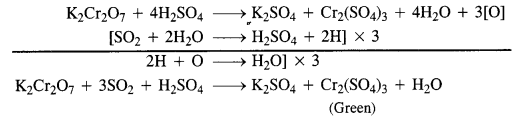Question 23.
Mention three areas in which H2SO4 plays an important role.
(i) It is used in the manufacture of fertilizers such as (NH4)2 SO4 , calcium superphosphate.
(ii)It is used as an electrolyte in storage batteries.
(iii)It is used in petroleum refining, detergent industry and in the manufacture of paints, pigments and dyes.

Question 24.
Write the conditions to maximise the yield of sulphuric acid by Contact process.
Catalytic oxidation of sulphur dioxide into sulphur trioxide. Sulphur dixocide is oxidised to sulphur trioxide with air in the presence of V2O5 or platinised asbestos acting as the catalyst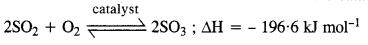Question 25.
Why is $${ K }_{ { a }_{ 2 } }$$ < $${ K }_{ { a }_{ 1 } }$$ for H2SO4 in water ?
H2SO4 is a very strong acid in water largely because of its first ionisation to H3O+ and HSO4– The ionisation of HSO4 to H3O+ and SO42- is very very small. That is why, $${ K }_{ { a }_{ 2 } }$$ < $${ K }_{ { a }_{ 1 } }$$.

Question 26.
Considering the parameters such as bond dissociation enthalpy, electron gain enthalpy and hydration enthalpy, compare the oxidising power of F2 and Cl2.
Fluorine is a better oxidising agent than chlorine because E°F2/F- is higher than E°Cl2/Cl- It is mainly due to low bond dissociation energy, high hydration energy and lower electron gain enthalpy, non-availability of d-orbitals in valence shell, that results in higher reduction potential of F2 than chlorine.

Question 27.
Give two examples to show the anomalous behaviour of fluorine.
The anomalous behaviour of fluorine, the first member of the halogen family as compared to the rest of the members is due to its very small size, very high electronegativity and absence of vacant d-orbitals in the valence shell. It is supported by the following points.
(i) Fluorine shows only negative oxidation state of -1 in its compounds. The other members exhibit both positive and negative oxidation states.
(ii) Fluorine forms hexafluoride with sulphur (SF6). No other member of the family forms hexahalide with sulphur.

Question 28.
Sea is the greatest source of some halogens. Comment.
The name halogen is a Greek Word meaning lsea salt forming’. Sea is a major source of a members of halogens particularly chlorine, bromine and iodine and they exist as the soluble salts of sodium, potassium, calcium, magnesium etc. The deposits of dried up sea water contain sodium chloride and carnallite (KClMgCl2.6H2O). Sea weeds contain nearly 0-5 percent of iodine. Similarly Chile saltpeter contains about 0-2% of sodium iodate (NaIO3).

Question 29.
Give reason for the bleaching action of Cl2.
Bleaching by chlorine occurs in the presence of moisture. In fact, it releases nascent oxygen on reacting with HO which carries bleaching. Since the reaction cannot be reversed, the bleaching by chlorine is permanent.
Chlorine bleaches by oxidation Cl2 + H2O → HCl + HOCl → HCl + [O]
The nascent oxygen reacts with dye to make it colourless.Question 30.
Name two poisonous gases which can be prepared from chlorine gas.
Two poisonous gases are phosgene and mustard gas.

Question 31.
Why is ICl more reactive than I2 ? (C.B.S.E. Outside Delhi 2012)
The reactivity of ICl is due to its polar nature (I—Cl). Iodine (I2) being non-polar is comparatively less reactive chemically.

Question 32.
Why is helium used in diving apparatus?
A mixture of helium and oxygen does not cause pain due to very low solubility of helium in blood as compared to nitrogen.

Question 33.
Balance the equation : XeF6 + H2O → XeO2F2 +HF (H.P. Board 2013)Question 34.
Why has it been difficult to study the chemistry of radon ?
Radon (Rn) is a radioactive element with very short half period of 3-82 days. Therefore, it becomes quite difficult to study the details about the chemistry of the element.

NCERT EXERCISE

Question 1.
Discuss the general characteristics of group 15 elements with reference to their electronic configuration, oxidation state, atomic size, ionisation enthalpy and electronegativity.
(i) Electronic configuration: All the elements in group 15 have 5 valence electrons. Their general electronic configuration is ns2np3

(ii) Oxidation states:
All these elements have 5 valence electrons and require three more electrons to complete octets. However, gaining electrons is very difficult as the nucleus will have to attract three more electrons. This can take place only with nitrogen as it is the smallest in size and the distance between the nucleus and the valance shell is relatively small. The remaining elements of this group show a formal oxidation state of -3 in their covalent compounds.

In addition to the -3 state, N and P also show -1 and.-2 oxidation states. All the elements present in this group show +3 and +5 oxidation states. However, the stability of the +5 oxidation state decreases down the group, whereas the stability of+3 oxidation state increases. This happens because of the inert pair effect.

(iii) Ionization energy and electronegativity First ionization energy decreases on moving down a group. This is because of increasing atomic sizes. As we move down a group, electronegativity decreases, owing to an increase in size.

(iv) Atomic size:
On moving down a group, the atomic size increases. This increase in the atomic size is attributed to an increase in the number of shells.

Question 2.
Why does the reactivity of nitrogen differ from phosphorus?
N2 exist as a diatomic molecule containing triple bond between two N-atoms. Due to the presence —of the triple bond between p  the two N-atoms, the bond dissociation energy is large (941 .4 kJ mol-1 ). As a result of this N2 is inert and unreactive whereas, phosphorus exists as a tetratomic molecule, containing P – P single bond. Due to the presence of single bond, the bond dissociation energy is weaker (213 kJmol-1 ) than N a N triple bond (941 .4 kJ mol-1 ) and moreover due to presence of angular strain in P4 tetrahedra. As a result of this, phosphorus is much more reactive than nitrogen.

Question 3.
Discuss the trends in chemical reactivity of group 15 elements.
(a) Hydrides: The hybrids are covalent with pyramidal structures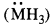and the central atom is sp3 hybrilised. The presence of the lone pair of electrons on the central atom distorts the geometry of the molecules and the bond angle less than that of a regular tetrahedron.(b) Halides:
Elements of group 15 form two types of halides viz. trihalides and pentahalides. The halides are predominantly basic (Lewis bases) in nature and have lone pair of electrons (central atom is sp3 hybridized). The pentahalides are thermally less stable than the trihalides.

(c) Oxides: All the elements of this group form two types of oxides ie., M2O3 and M2O5 and are called trioxides and pentoxides

Question 4.
Why does NH3 form hydrogen bonding while PH3 does not?
The N—H bond in ammonia is quite polar on account of the electronegativity difference of N (3·0) and H (2·1). On the contrary, P—H bond in phosphine is almost non-polar because both P and H atoms have almost same electronegativity (21). Due to polarity, intermolecular hydrogen bonding is present in the molecules of ammonia but not in those of phosphine.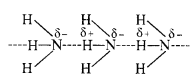Question 5.
How is nitrogen prepared in the laboratory? Write the chemical equations of the reactions involved.
Laboratory preparation: Dinitrogen is prepared in the laboratory by heating a solution containing an equivalent amount of sodium nitrite and ammonium chloride.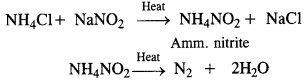Question 6.
How is ammonia manufactured industrially?
Ammonia is prepared on a commercial scale by Haber’s Process from dinitrogen and dihydrogen by the following chemical reaction
N2 + 3H2 ⇌ 2NH3; ∆fH° = – 46.1 kJ mon-1
Dihydrogen needed for the commercial preparation of ammonia is obtained by the electrolysis of water while dinitrogen is obtained from the liquefied air as a fractional distillation. The two gases are purified and also dried.
These are compressed to about 200 atmosphere pressure and are then led into the catalyst chamber packed with the catalyst and the promoter.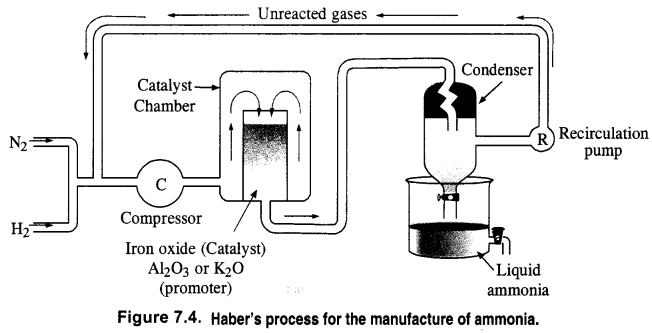Question 7.
Illustrate how copper metal gives different products on reaction with HNO3.
Anhydrous nitric acid is colourless, fuming, and pungent-smelling liquid. However, it acquires a yellowish-brown colour in the presence of sunlight. HNO3 decomposes to give NO2 gas which dissolves and imparts its yellowish-brown colour.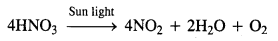On strong heating, the acid decomposes to give NO2 and O2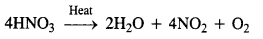Question 8.
Give the resonating structures of NO2 and N2O5.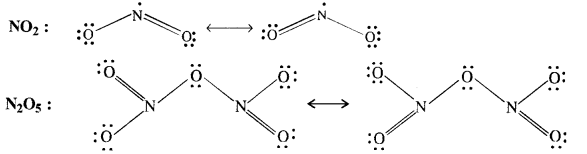Question 9.
The HNH angle value is higher than those of HPH, HAsH, and HSbH angles; why?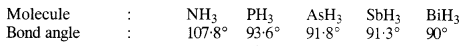The above trend in the H-M-H bond angle can be explained on the basis of the electronegativity tif central atom. Since nitrogen is highly electronegative, there is high electron density around nitrogen. This causes greater repulsion between the electron pairs around nitrogen, resulting in maximum bond angle. We know that electronegativity decreases on moving down a group consequently. The repulsive interactions between the electron pairs decrease, thereby decreasing the HMH bond angle.

Question 10.
Why does R3P = O exist but R3N = O does not (R = alkyl group)?
Nitrogen does not contain d-orbitals. As a result, it cannot expand its covalency beyond four and cannot form pπ – dπ multiple bonds. In contrast, P contains the d-orbitals, and can expand its covalency beyond 4 and can form pπ-dπ multiple bonds. Hence R3P = O exist but R3N = O does not.

Question 11.
Explain why is NH3 basic while PH3 is feebly basic in nature. (C.B.S.E. Outside Delhi 2008, 2009, Jharkhand Board 2009)
NH3 is distinctly basic while BiH3 is feebly basic.
Nitrogen has a small size due to which the lone pair of electrons are concentrated in a small region. This means that the charge density per unit volume is high. On moving down a group, the size of the central atom increases, and the charge gets distributed over a large area decreasing the electron density. Hence the electron-donating capacity of group 15 elements hydrides decreases on moving down the group.

Question 12.
Nitrogen exists as a diatomic molecule (N2) while phosphorus a tetra-atomic molecule (P4). Why?
Nitrogen is diatomic gaseous molecule at ordinary temperature due to its ability to form pπ – pπ multiple bonds. The molecule has one σ and two π – bonds. Phosphorus exists as discrete tetratomic tetrahedral molecules as these are not capable of forming multiple bonds due to repulsion between non-bonded electrons of the inner core.

Question 13.
Write the main difference between the properties of white and red phosphorus. (C.B.S.E. Delhi 2012)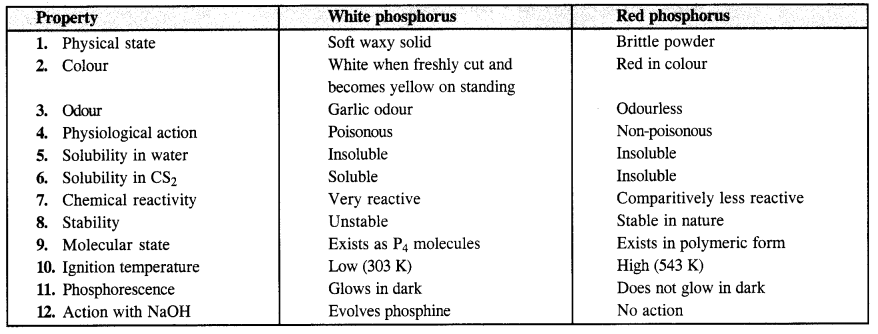Question 14.
Why does nitrogen show catenation properties less than phosphorus?
The catenation properties depend upon the strength of the element-element bond. The N-N bond strength is much weaker (due to the repulsion of lone pairs on nitrogen because of its small size) than the P-P bond strength, therefore, nitrogen shows catenation less than phosphorus.

Question 15.
Give one disproportionation reaction of phosphorus acid (H3PO3).
Upon heating to about 573 K, phosphorus acid undergoes disproportionation as follows :Question 16.
Can PCl5 act as oxidising as well as a reducing agent? Justify.
In general, the molecules of a substance can behave as a reducing agent if the central atom is in a position to increase its oxidation number. Similarly, they can act as an oxidising agent if the central atom is in a position to decrease its oxidation number. Now, the maximum oxidation state or oxidation number of phosphorus (P) is +5. It cannot increase the same but at the same time can decrease its oxidation number. In PCl5, oxidation number of P is already +5. It therefore, cannot act as a reducing agent. However, it can behave as an oxidising agent in certain reactions in which its oxidation number decreases. For example,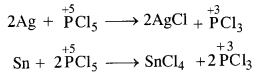Question 17.
Justify the placement of O, S, Se, Te and Po in the same group of the periodic table in terms of electronic configuration, oxidation states and hydride formation.
The members of the oxygen family are placed in group 16 of p-block. Their inclusion in the same group is justified on the basis of the following characteristics.
1. Electronic configuration. Members of the family have ns2p4 configuration. Their group (10 + 6) is 16.
2. Oxidation states. With exception of oxygen which exhibits -2 oxidation state in its compounds (OF2 and H2O2 are exceptions), rest of the members of the family show variable oxidation states (-2, +2, +4, +6) in their compounds.
3. Hydride formation. All the members of the family form covalent hydrides (MH2) in which the central atom is sp3 hybridised. These have angular structures and their characteristics show regular gradation.FeS + H2SO4 (dil.) → FeSO4 + H2S
Na2Se + H2SO4 (dil.) → Na2SO4 + H2Se

Question 18.
Why is dioxygen a gas while sulphur is a solid? (C.B.S.E. Delhi 2013)
The oxygen atom has a tendency to form pπ-pπ multiple bonding due to its small size and high electronegativity. As a result, oxygen exists as a diatomic molecule. These mol¬ecules are held together by weak van der Waal’s forces of attraction which can be easily overcome by collisions of the molecules at room temperature. Therefore, O2 is the gas at room temperature.

Sulphur, on the other hand, because of its bigger size and lower electronegativity, does not form pπ-pπ multiple bonds. Instead, it prefers to form an S-S single bond and form polyatomic complex molecules having eight atoms per molecule (S8) and have puckered ring structure. Therefore, S atoms are strongly held together and it exists as a solid.

Question 19.
Knowing the electron gain enthalpy values for O → O and O → O2- as – 141 kJ mol-1 and 702 kJ mol-1 respectively, how can you account for the formation of a large number of oxides having O2- species and not O ?
The stability of an ionic compound depends on its lattice energy. The more the lattice energy of a compound, the more stable it will be. Lattice energy is directly proportional to the charge carried by an ion. When a metal combines with oxygen, the lattice energy of the oxide involving O2- ion is much more than the oxide involving O ion. Hence, we can say that the formation of O2- is energetically more favourable than the formation of O

Question 20.
Which aerosols deplete ozone?
Aerosols or chlorofluorocarbons (CFC’s) such as freon (CCl2F2) deplete ozone layer by supplying chlorine-free radicals (Cl) which convert ozone into oxygen.Question 21.
Describe the manufacture of H2SO4 by Contact process.
Sulphuric acid is manufactured by the Contact process which involves three steps :

1. burning of sulphur or sulphide ores in air to generate SO2.
2. conversion of SO2 to SO3 by the reaction with oxygen in the presr nee of a catalyst (V2O5), and
3. absorption of SO3 in H2SO4 to give oleum (H2S2O7).

A flow diagram for the manufacture of sulphuric acid is shown in the figure. The SO2 produced is purified by removing dust and other impurities such as arsenic compounds. The key step in the manufacture of H2SO4 is the catalytic oxidation of SO2 with O2 to give SO3 in the presence of V2O5 (catalyst).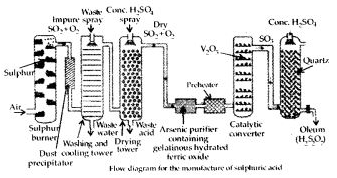The reaction is exothermic, reversible and the forward reaction leads to a decrease in volume. Therefore, low temperature and high pressure are favourable conditions for maximum yield. But the temperature should not be very low otherwise rate of reaction will become slow.

In practice, the plant is operated at a pressure of 2 bar and a temperature of 720 K. The SO3 gas from the catalytic converter is absorbed in concentrated H2SO4 to produce oleum. Dilution of oleum with water gives H2SO4 of the desired concentration. In the industry, two steps are carried out simultaneously to make the process a continuous one and also to reduce the cost.

Question 22.
How is SO2 an air pollutant?

• It combines with water vapour present in the atmosphere to form sulphuric acid. This causes acid rain. Acid rain damages soil, plants and buildings, especially those made of marble.
• Even in very low concentrations, SO2 causes irritation in the respiratory tract. It causes throat and eye irritation and can also affect the larynx to cause breathlessness.
• It is extremely harmful to plants. Plants exposed to sulphur dioxide for a long time lose colour from their leaves. This condition is known as chlorosis. This happens because the formation of chlorophyll is affected by the presence of SO2.

Question 23.
Why are halogens strong oxidising agents?
The general electronic configuration of halogens is np5, where n = 2-6. Thus, halogens need only one more e- to complete their octet and to attain the stable noble gas configuration. Also, halogens are highly electronegative with low dissociation energies and high negative electron gain enthalpies. Therefore, they have a high tendency to gain an election. Hence, they act as strong oxidizing agents.

Question 24.
Explain why does fluorine form only one oxoacid (HOF).
The members of the halogen family with the exception of fluorine show variable oxidation states due to the availability of rf-orbitals for the bond formation. They form a number of oxoacids such as HOX, HOXO, HOXO2, and HOXO3. However, fluorine which is highly electronegative and has no rf-orbitals, forms only one oxoacid (HOF) in which its oxidation state is +1.

Question 25.
Explain why in spite of nearly the same electronegativity, nitrogen is involved in hydrogen bonding while chlorine is not.
Both chlorine and oxygen have almost the same electronegativity values, but chlorine rarely forms hydrogen bonding. This is because in comparison to chlorine, oxygen has a smaller size and as a result, a higher electron density per unit volume.

Question 26.
Write two uses of ClO2.
ClO2 is a strong oxidising agent. Therefore,
(i) It acts as bleaching agent for paper pulp in paper industry and in textile industry.
(ii) It acts as germicide for disinfecting water.

Question 27.
Why are halogens coloured?
All the halogens are coloured in nature. The colour deepens with the increase in the atomic number of the element from fluorine to iodine.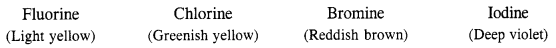The cause of the colour is due to the absorption of energy from the visible light by the molecules for the excitation of outer non -bonded electrons to higher energy levels. The excitation energy depends upon the size of the atom. Fluorine has the smallest size and the force of attraction between the nucleus and electrons is very large.

Question 28.
Write the reactions of F2 and Cl2 with water.Question 29.
How can you prepare Cl2 from HCl and HCl from Cl2? Write chemical equations only.
(i) HCl can be oxidised to chlorine with the help of a number of oxidising agents like MnO2, KMnO4, K2Cr2O7 etc.
Mn0O2 + 4HCl → MnCl2 + Cl2 + 2H2O
(ii) Cl2 can be reduced to HCl by reacting with H2 in the presence of sunlight. The gas on passing through water dissolves to form hydrochloric acidQuestion 30.
What inspired N. Bartlett for carrying out the reaction between Xe and PtF6?
The X-ray study of the compound has shown it be a crystalline solid consisting of O2+ and (PtF6] ions. In this reaction, PtF6 has oxidised O2 to O2+ ion. Bartlett through than PtF6 should Xe to xe+ since first ionisation enthalpy of xenon (1176 kJ mol-1) is quite close to that of O2 (1180 kJ mol-1).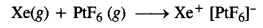Question 31.
What is the oxidation state of phosphorus in the following?
(a) H3PO3
(b) PCl3
(c) Ca3P2
(d) Na3PO4
(e) POF3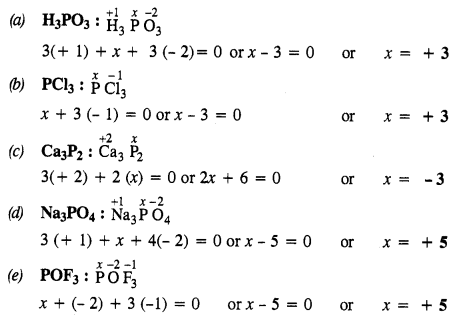Question 32.
Write balanced equations for the following :
(i) NaCl is heated with sulphuric acid in the presence of MnO2
(iii) Chlorine gas is passed into a solution of Nal in water.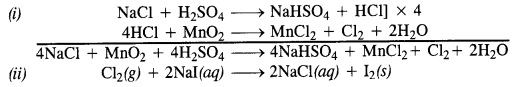Question 33.
How are xenon fluorides XeF2, XeF4 and XeF6 obtained ?
Xenon forms three binary fluorides, XeF2, XeF4 and XeF6 by the direct reaction of elements under appropriate experimental conditions.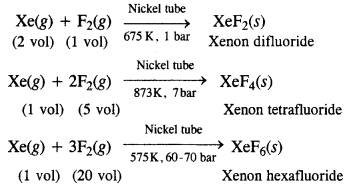Question 34.
With what neutral molecule is ClO isoelectronic? Is that molecule a Lewis base?
OF2 and ClF are isoelectronic to ClO, out of which ClF is a Lewis base.

Question 35.
How are XeO3 and XeOF4 prepared?
Preparation of XeO3. By complete hydrolysis of XeF6.
XeF6 + 3H2O → XeO3+ 6HF.
Preparation ofXeOF4. By partial hydrolysis of XeF6.
XeF6 + H2O → XeOF4+ 2HF.

Question 36
Arrange the following in the order of property indicated for each set:
(i) F2, Cl2, Br2, I2 – increasing bond dissociation enthalpy.
Bond dissociation energy usually decreases on moving down a group as the atomic size increases. However, the bond dissociation energy of F2 is lower than that of Cl2 and Br2. This is due to the small atomic size of fluorine, Thus, the increasing order for bond dissociation energy among halogens is as follows:
I2 < F2 < Br2 < Cl2

(ii) HF, HCl, HBr, HI – increasing acid strength.
HF < HCl < HBr < HI
The bond dissociation energy of HX molecules where X – F, Cl, Br, I, decreases with an increase in the atomic size. Since H – I bond is the weakest, HI is the strongest acid.

(iii) NH3, PH3, ASH3, SbH3, BiH3 – increasing base strength.
BiH3 < SbH3 < AsH3 < PH3 < NH3 On moving from nitrogen to bismuth, the size of the atom increases while the electron density on the atom decreases. Thus, the basic strength decreases.

Question 37.
Which one of the following does not exist?
(i) XeOF4
(ii) NeF2
(iii) XeF2
(iv) XeF6.
NeF2 does not exist because the element Ne(Z = 10) with 1s22s22p6 does not have vacant 2d orbitals. As such, there is no scope of any electron promotion even by a highly electronegative element.

Question 38.
Give the formula and describe the structure of a noble gas species which is isostructural with :
(i) $$IC{ l }_{ 4 }^{ – }$$
(ii) $$IB{ r }_{ 2 }^{ – }$$
(iii) $$Br{ O }_{ 3 }^{ – }$$(i) Structure of $$IC{ l }_{ 4 }^{ – }$$. The central I atom has in all 8 electrons (7 valence electrons +1 due to negative charge). Out of these, it shares 4 electrons with four atoms of Cl and the remaining four electrons constitute two lone pairs. In all, there are six pairs. The structure of the ion must be octahedral or distorted square planar in order to minimise the forces of repulsion among the two electrons pairs. $$IC{ l }_{ 4 }^{ – }$$ has (7 + 4 x 7 + 1) = 36 valence electrons and it iso-electronic and iso-structural with XeF4 (8 + 4 x 7) which has also 36 valence electrons.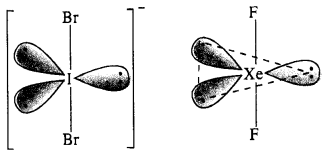(ii) Structure of $$IB{ r }_{ 2 }^{ – }$$.
In $$IB{ r }_{ 2 }^{ – }$$ ion, the central I atom has 8 valence electrons (7 + 1). Out of these, it shares 2 electrons with two atoms of Br and the remaining 6 electrons constitute three lone pairs. In all, there are five pairs. The structure of the ion must be trigonal bipyramidal or linear in order to minimise the force of repulsion among the three lone electrons pairs. IBrf has (7 + 2 x 7 + 1) = 22 valence electrons and is isoelectronic as well as iso-structural with noble gas species XeF2 which has also 22 (8 + 2 x 7) electrons.(iii) Structure of $$Br{ O }_{ 3 }^{ – }$$ ion. In $$Br{ O }_{ 3 }^{ – }$$ ion, the central Br atom has 8 valence electrons (7 + 1). Out of these, it shares 4 with two atoms of O forming Br=0 bonds. Out of the remaining four electrons, 2 are donated to the third O atom and account for its negative charge. The remaining 2 electrons constitute one lone pair. In order to minimise the force of repulsion, the structure of $$Br{ O }_{ 3 }^{ – }$$ ion must be pyramidal. $$Br{ O }_{ 3 }^{ – }$$ ion has (7 + 3 x 6 + 1) = 26 valence electrons and is isoelectronic as well as iso-structural with noble gas species Xe03 which has also 26 (8 + 3 x 6) electrons.

Question 39.
Why do noble gases have comparatively large atomic sizes?
Noble gases do not form molecules. In the case of noble gases. the atomic radii correspond to Van der Waa1s radii. On the other hand, the atomic radii of other elements correspond to their covalent radii. By definition, Yan der Waal’s radii are larger than covalent radii. It is for this reason that noble gases are very large in size as compared to other atoms belonging to the same period.

Question 40.
List the uses of neon and argon gases.
Uses of Neon: